# Plot a trajectory on dimensionality reduction

Plot a trajectory on dimensionality reduction

```plot_dimred(trajectory, color_cells = c("auto", "none", "grouping",
"feature", "milestone", "pseudotime"),
dimred = ifelse(dynwrap::is_wrapper_with_dimred(trajectory), NA,
ifelse(length(trajectory\$cell_ids) > 500, dimred_pca, dimred_mds)),
plot_trajectory = dynwrap::is_wrapper_with_trajectory(trajectory) &&
!plot_milestone_network, plot_milestone_network = FALSE,
label_milestones = dynwrap::is_wrapper_with_milestone_labelling(trajectory),
alpha_cells = 1, size_trajectory = 1, grouping = NULL,
groups = NULL, feature_oi = NULL, color_milestones = c("auto",
"given", "cubeHelix", "Set3", "rainbow"), milestones = NULL,
milestone_percentages = NULL, pseudotime = NULL,
expression_source = "expression", color_density = c("none",
"grouping", "feature"), padding = 0.1, nbins = 1000, bw = 0.2,
density_cutoff = 0.3, density_cutoff_label = density_cutoff/10,
waypoints = dynwrap::select_waypoints(trajectory),
trajectory_projection_sd = sum(trajectory\$milestone_network\$length) *
0.05, color_trajectory = "none")```

## Arguments

trajectory The trajectory How to color the cells The dimensionality reduction matrix (with cell_ids as rownames) or function which will run the dimensionality reduction Whether to plot the projected trajectory on the dimensionality reduction Whether to plot the projected milestone network on the dimensionality reduction How to label the milestones. Can be TRUE (in which case the labels within the trajectory will be used), "all" (in which case both given labels and milestone_ids will be used), a named character vector, or FALSE The alpha of the cells The size of the trajectory segments The grouping of the cells Tibble containing information of the cell groups feature to plot expression How to color the cells Tibble containing the `milestone_id` and a `color` for each milestone The milestone percentages The pseudotime Source of the expression How to color density, can be "none", "grouping", or "feature" The padding in the edges to the plot, relative to the size of the plot Number of bins for calculating the density Bandwidth, relative to the size of the plot Cutoff for density, the lower the larger the areas Cutoff for density for labelling, the lower the further way from cells The waypoints to use for projecting, as generated by [dynwrap::select_waypoints()] The standard deviation of the gaussian kernel to be used for projecting the trajectory. This is in the order of maginature as the lengths of the milestone_network. The lower, the more closely the trajectory will follow the cells How to color the trajectory, can be "nearest" for coloring to nearest cell, or "none"

## Examples

```data(example_bifurcating)
plot_dimred(example_bifurcating)#> Coloring by milestone#> Using milestone_percentages from trajectory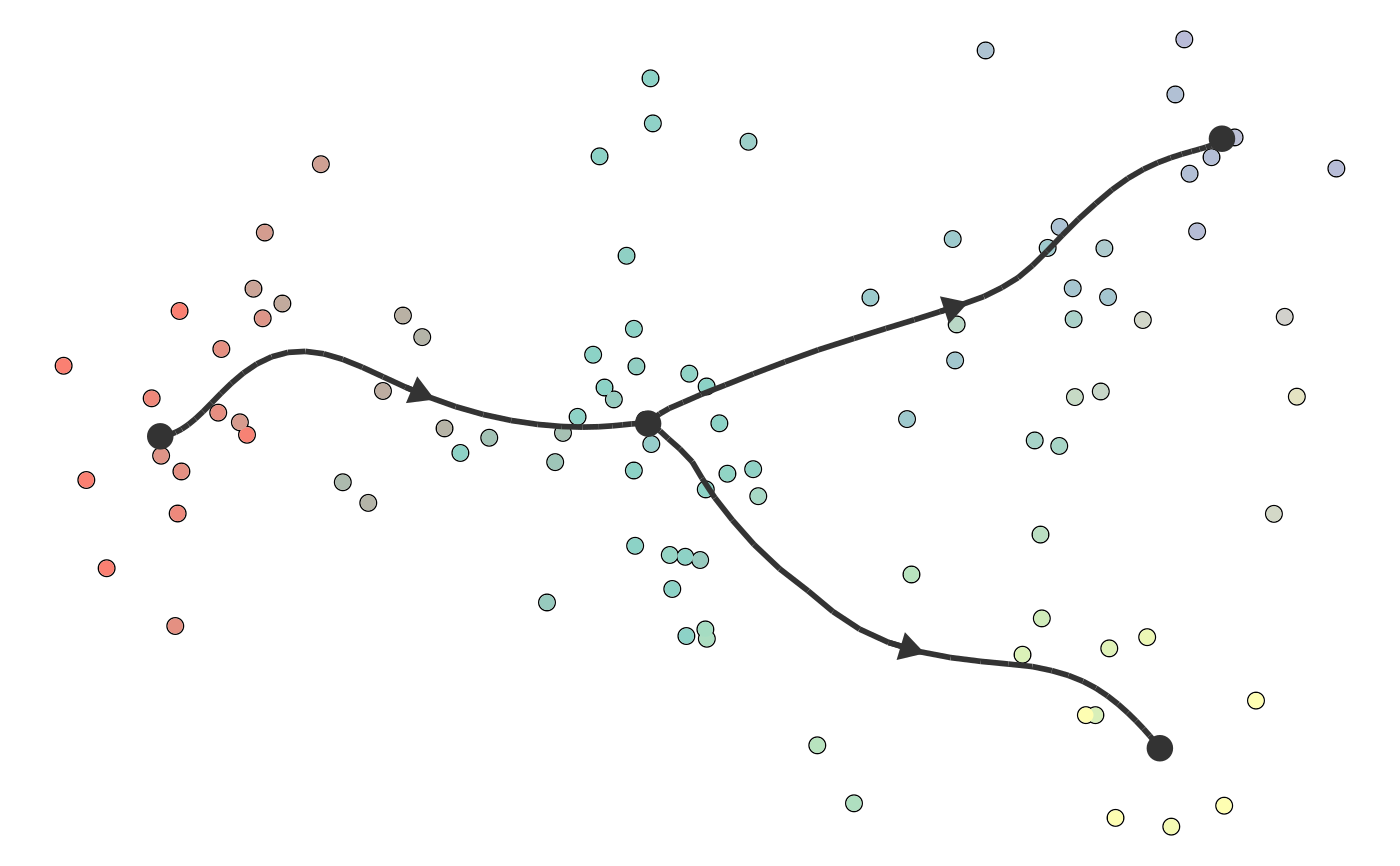plot_dimred(example_bifurcating, dimred = dyndimred::dimred_umap)#> Coloring by milestone#> Using milestone_percentages from trajectory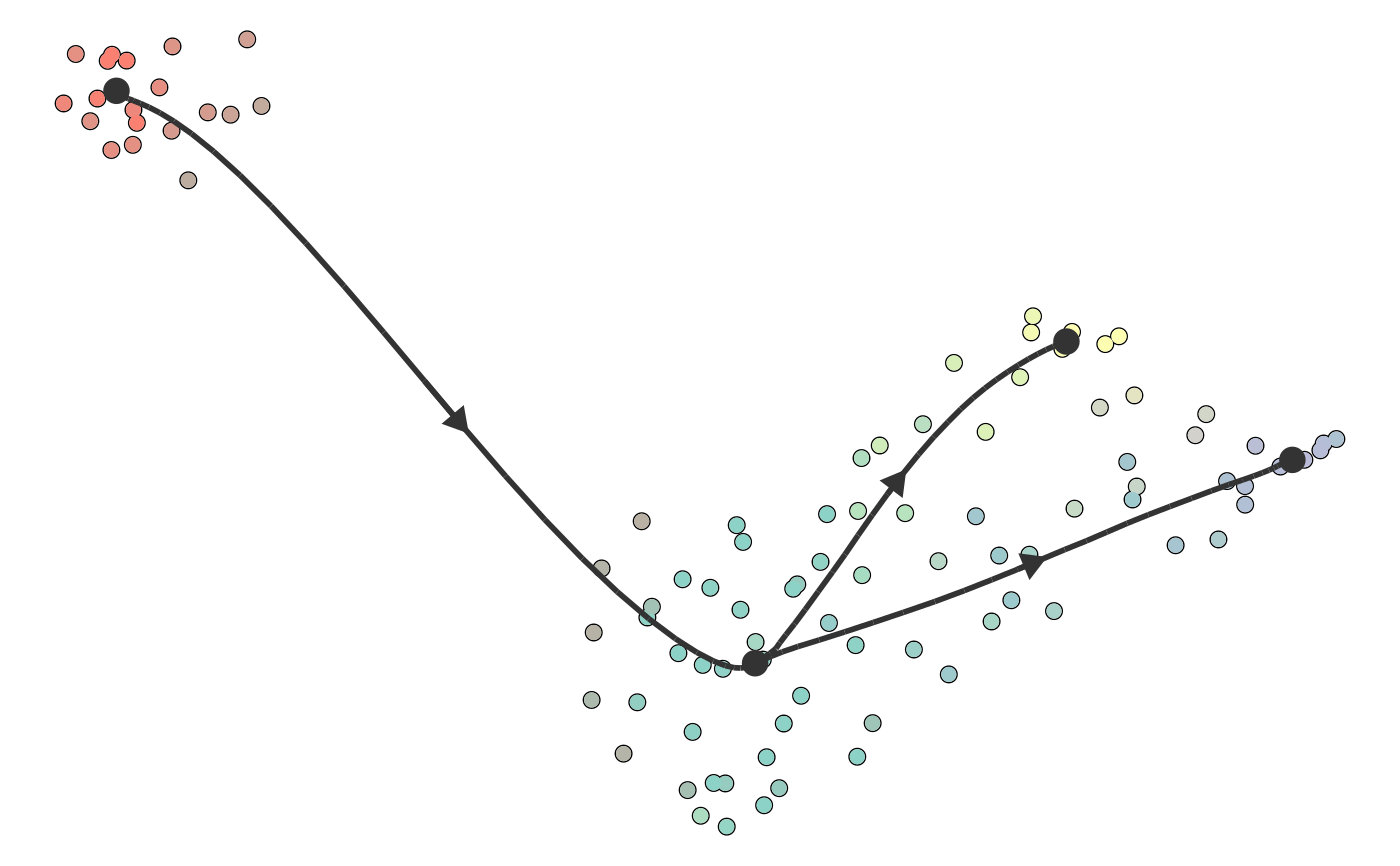dimred <- dyndimred::dimred_dm_destiny(example_bifurcating\$expression)
plot_dimred(example_bifurcating, dimred = dimred)#> Coloring by milestone#> Using milestone_percentages from trajectory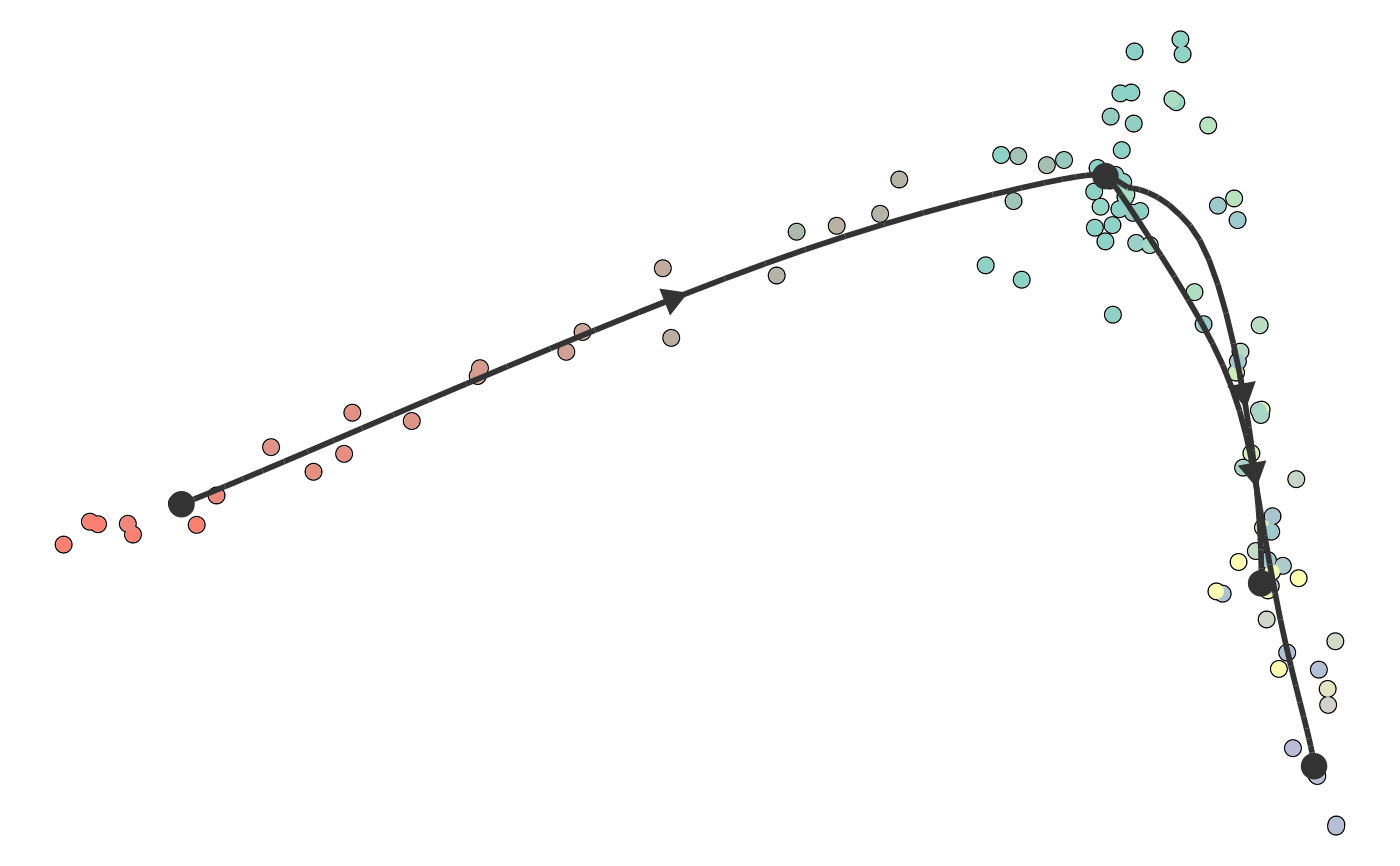plot_dimred(example_bifurcating, color_cells = "pseudotime")#> Pseudotime not provided, will calculate pseudotime from root milestone#> root cell or milestone not provided, trying first outgoing milestone_id#> Using 'M1' as root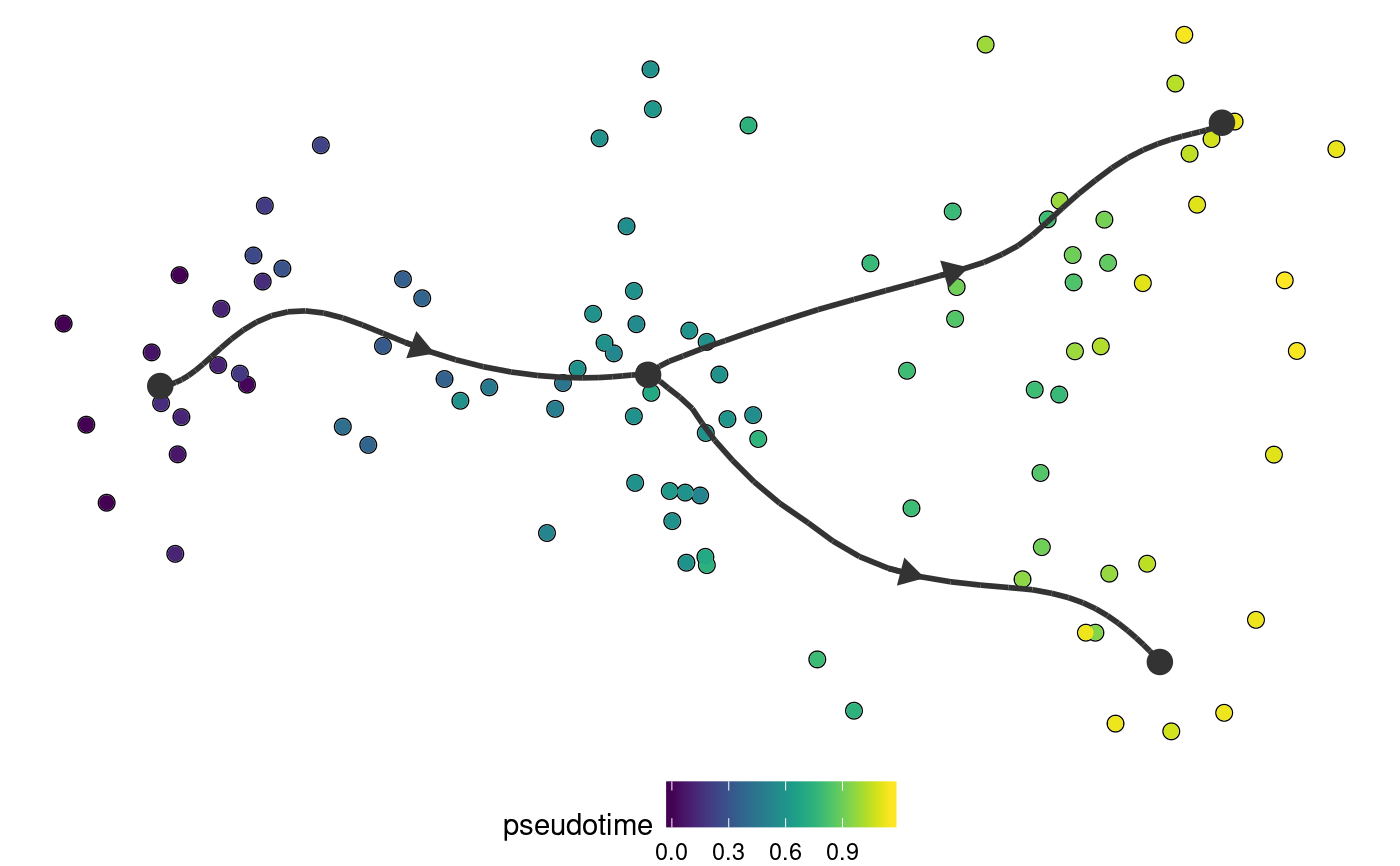plot_dimred(
example_bifurcating,
color_density = "grouping",
grouping = dynwrap::group_onto_nearest_milestones(example_bifurcating)
)#> Coloring by grouping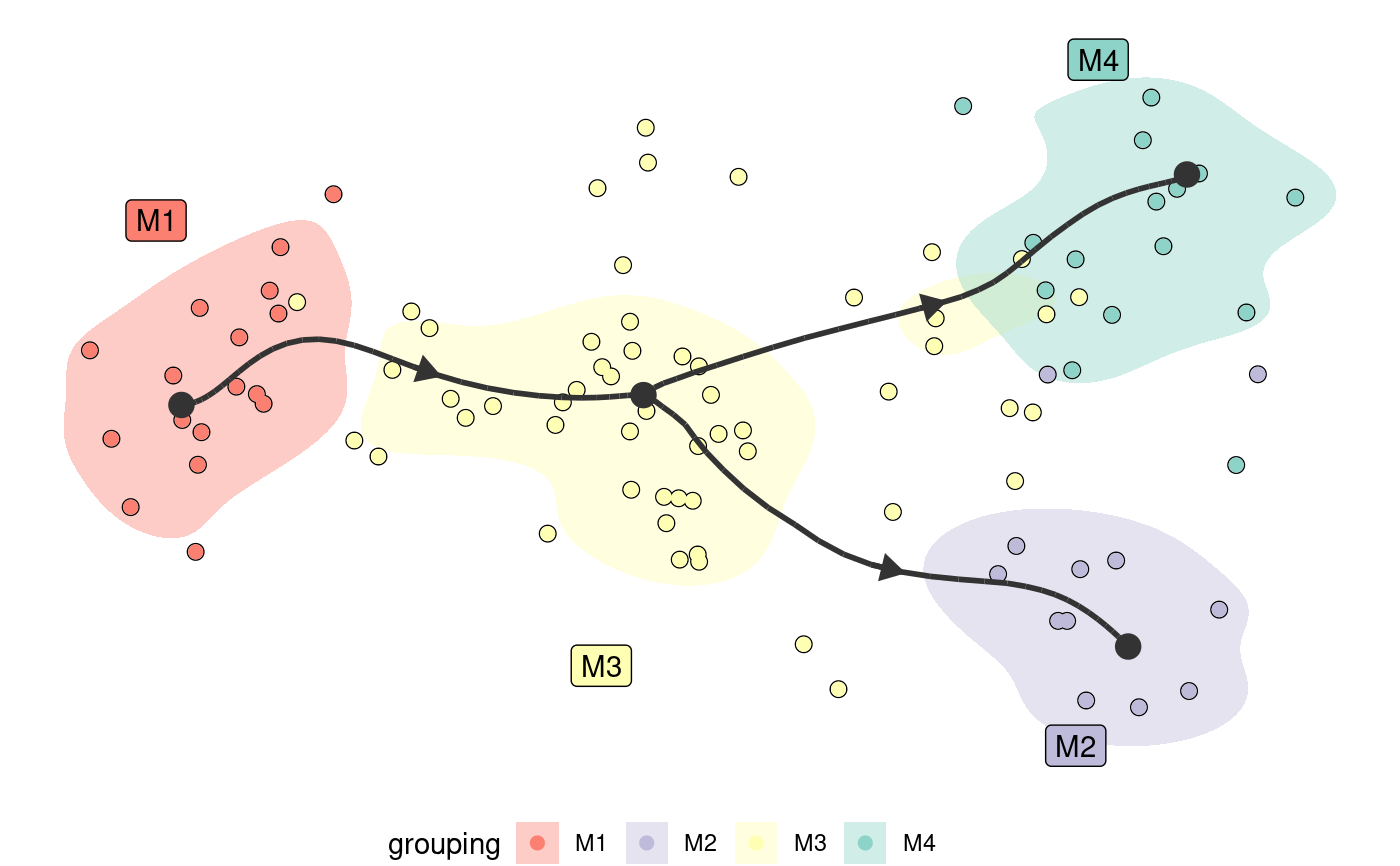```